# Congruent Triangles And Their Properties

In this quiz, various multiple-choice questions related to the congruent triangles and their properties are asked. The students will be required to check if two triangles are congruent or not as well well they would be required to find the lengths of the triangles.

Start Quiz

Two figures are said to be congruent, if they have exactly the same:

length and width

shape and size

area

perimeter

Choose the correct option given two triangles are congruent.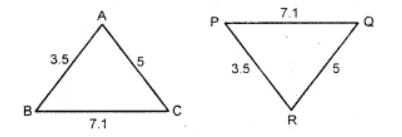Triangle ABC is congruent to triangle QRP

Triangle ABC is congruent to triangle PQR

Triangle ABC is congruent to triangle RPQ

Are the given triangles congruent?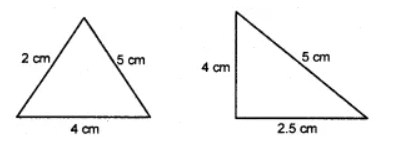yes

no

Are the given triangles congruent?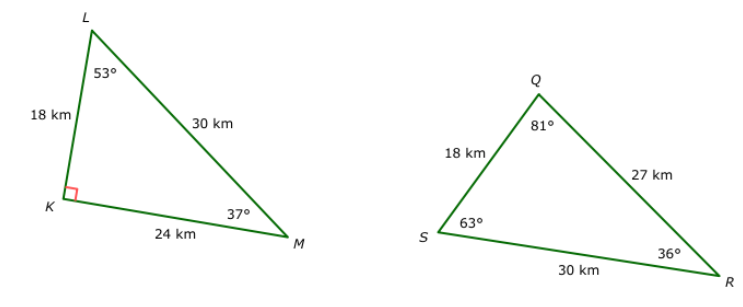yes

no

Are these given figures similar?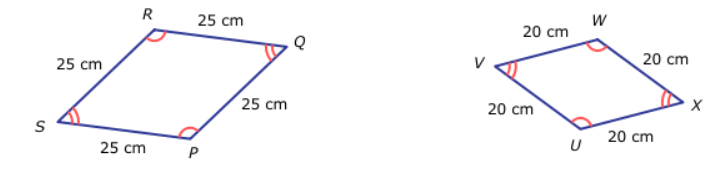yes

no

If the given triangles are congruent, find the length of LN.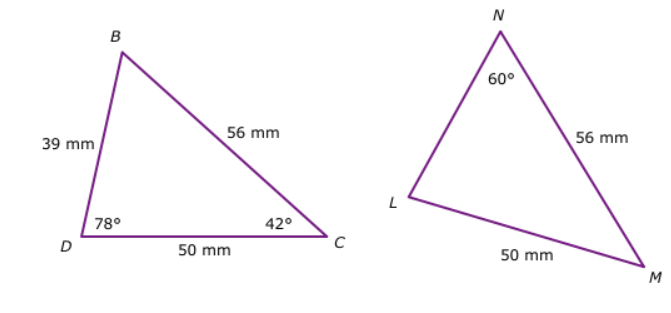LN = ##39## millimeters

39

If the given triangles are congruent, find the angle NLM.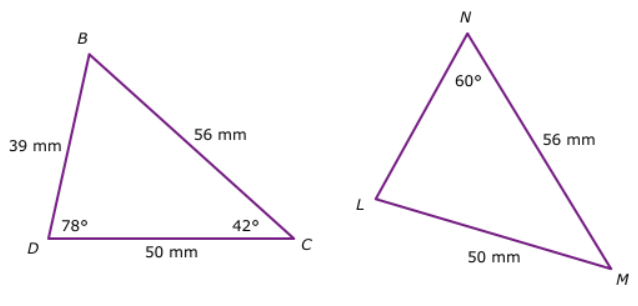Angle NLM = ##78## degrees

78

If the given triangles are congruent, find the length of LM.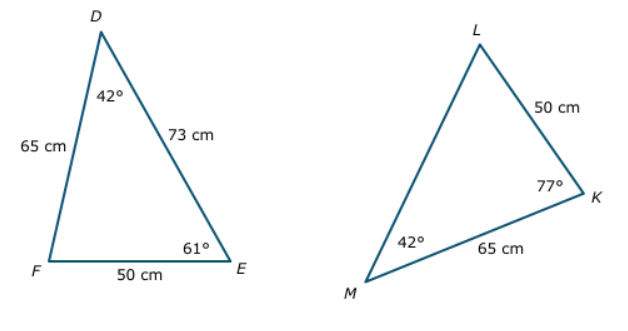LM = ##73## centimeters

73
Quiz/Test Summary
Title: Congruent Triangles And Their Properties
Questions: 8
Contributed by: